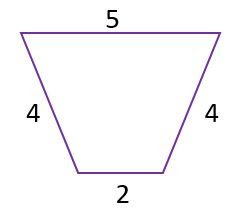# High School Geometry: Help and Review Final Exam

Free Practice Test Instructions:

Choose your answer to the question and click 'Continue' to see how you did. Then click 'Next Question' to answer the next question. When you have completed the free practice test, click 'View Results' to see your results. Good luck!

#### Question 2 2. Locate point (-2, 2).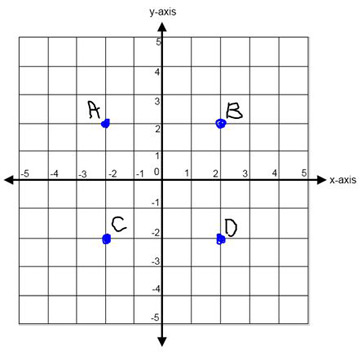#### Question 3 3. Which pair of angles are alternate interior angles?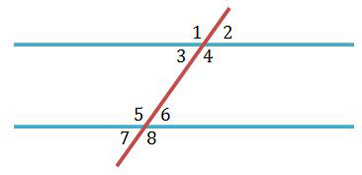#### Question 4 4. All of the following statements about the pictured triangle must be true, EXCEPT: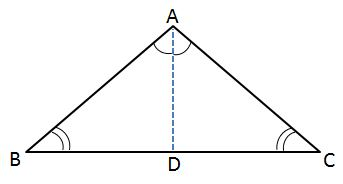#### Question 5 5. If arc AC is 122 degrees and arc BC is 192 degrees, what is the measure of angle ACB?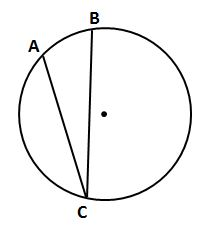#### Question 6 6. Given the triangle PQE, calculate the measure of Angle Q.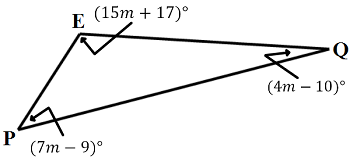#### Question 10 10. In the picture below, if arc AB is 82 degrees, what is the measure of angle BAC?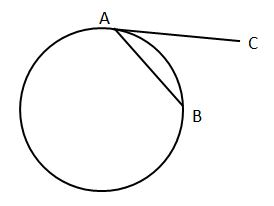#### Question 12 12. In the pictured triangle, XV is an angle bisector. What is the length of XY?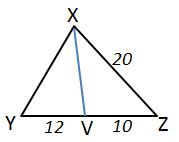#### Question 14 14. Find the perimeter.